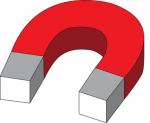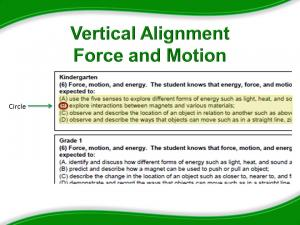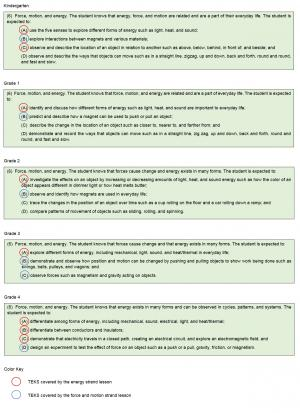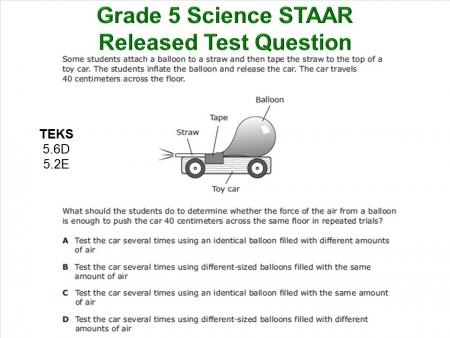# Force and Motion Strand Summary

Let's refer back to the Force and Motion Lesson Summaries page that you began using during the Force and Motion: Engage section.

How does the Grade 2–Force and Motion lesson build on the grade 1 and kindergarten lessons? How does it support the grade 3 and grade 4 lessons?

The kindergarten lesson focuses on magnetic and nonmagnetic materials. Grade 1 looks at how magnets push and pull. Grade 2 observes how magnets are used in everyday life. Grade 3 introduces gravity as a force, how to measure force using a spring scale, the concept of work, and simple machines. Grade 4 introduces the force of friction and how to design an experiment. Each grade level depends on the grade prior to lay the appropriate cognitive foundation. It is very important that we each do our part to help our students be successful.

# Force and Motion Main Idea

Let's refer back to the force, motion, and energy vertical alignment study. Write a main idea statement for each grade level as it relates to the science concept TEKS that were addressed by the force and motion lessons. Please label each statement with the correlating grade level.Force and Motion Strand Main Ideas

How do the lessons vertically align to support the learning of force and motion concepts?

Write one sentence that summarizes how the lesson addresses the targeted TEKS for each grade level in the force and motion strand. You should have a total of five sentences when finished. Please label each statement with the correlating grade level.

# Force and Motion Vertical Alignment

Identify the science concepts that are addressed by the force and motion lessons using the cover sheets from each lesson. Circle the letter of any highlighted TEKS on your TEKS chart.What do you observe about the TEKS you circled? Each of the lessons addresses TEKS that support high school physics. It may seem that as elementary educators, we are far removed from the world of a physics student. However, we lay the foundation and have a direct impact on the academic success of that student. By teaching these lessons, we have done our part to build and strengthen the knowledge of our students.

Scroll down to see which TEKS were addressed by the force and motion strand lessons.# Grade 5 STAAR Released Test Question

How does elementary science support the fifth-grade knowledge needed to answer this question?How do we prepare students to answer this question?

Notice that the question is dual coded for process skills 5 (2)(E) and content knowledge 5 (6)(D). The correct answer is C.

At the conclusion of this lesson, you may complete the Force and Motion Assessment.

# Force and Motion Assessment

Now take a moment to complete the Force and Motion Assessment below.

Interactive